Up

## Pythagoras Theorem With Animations Educational PowerPoint Template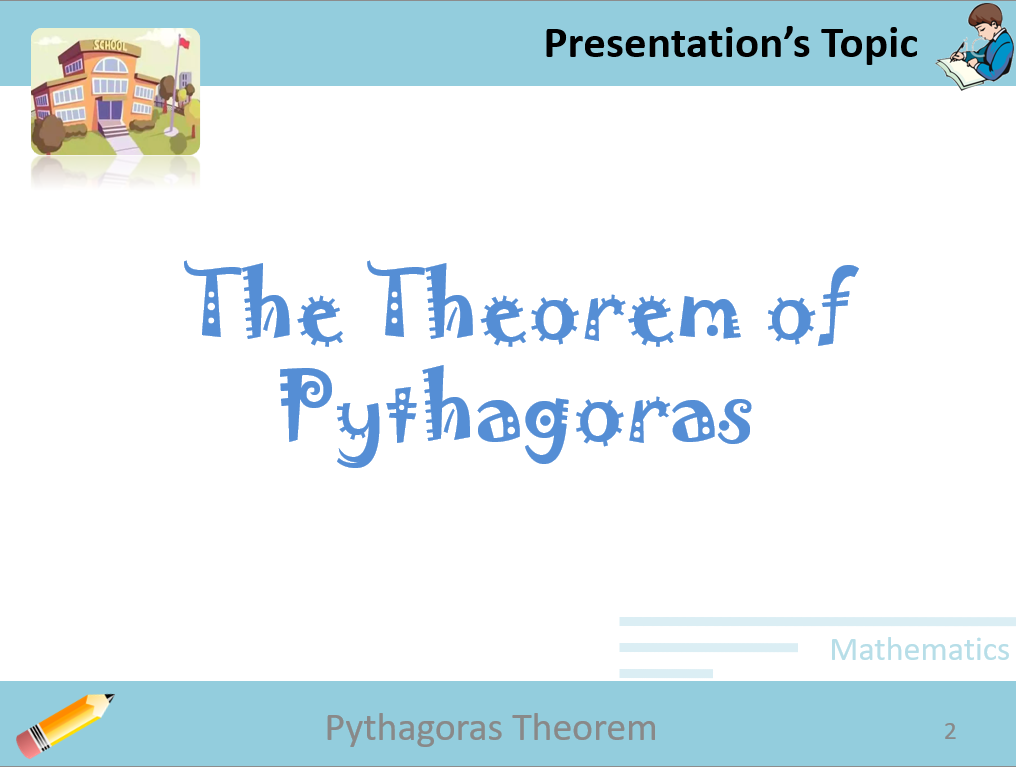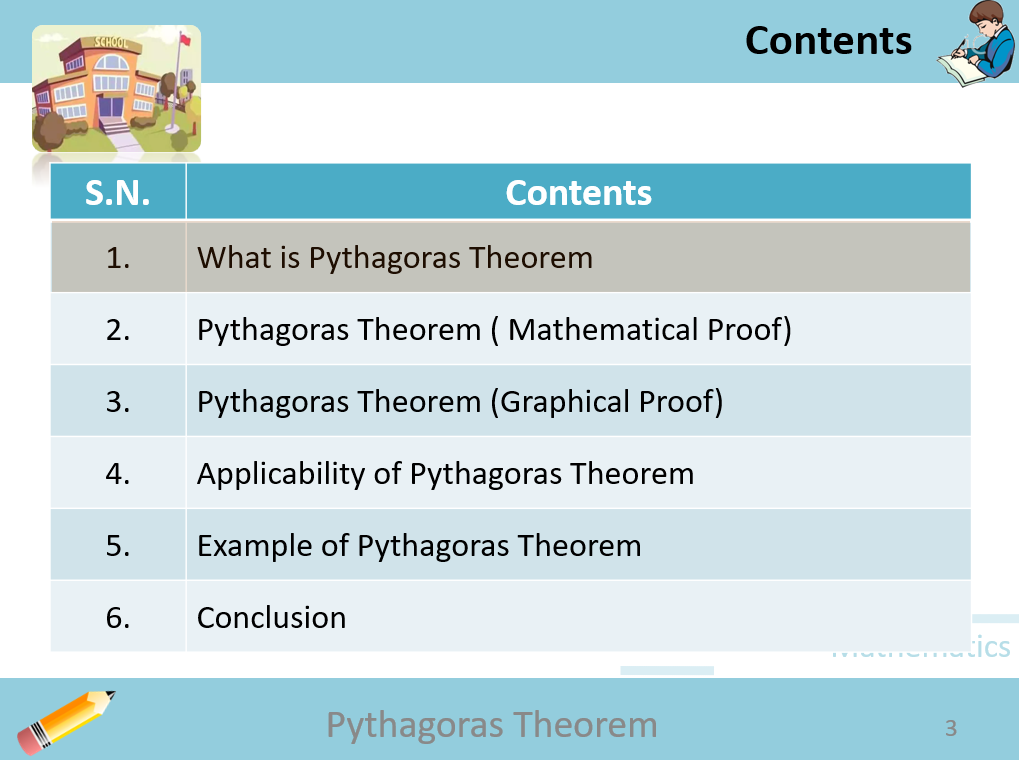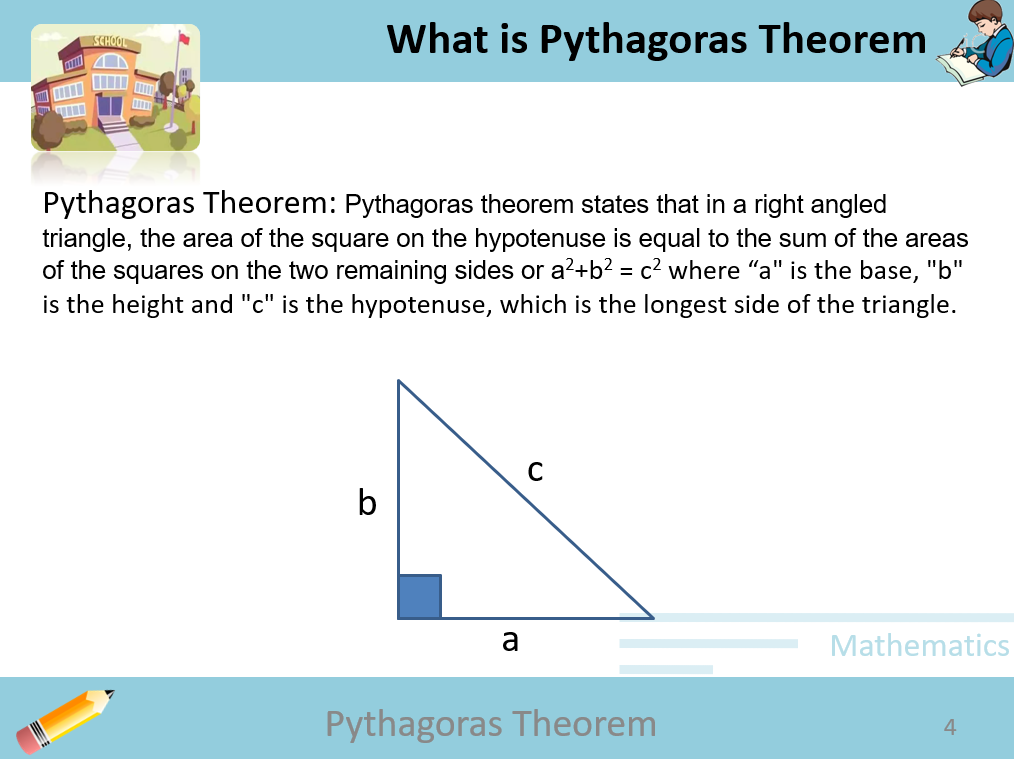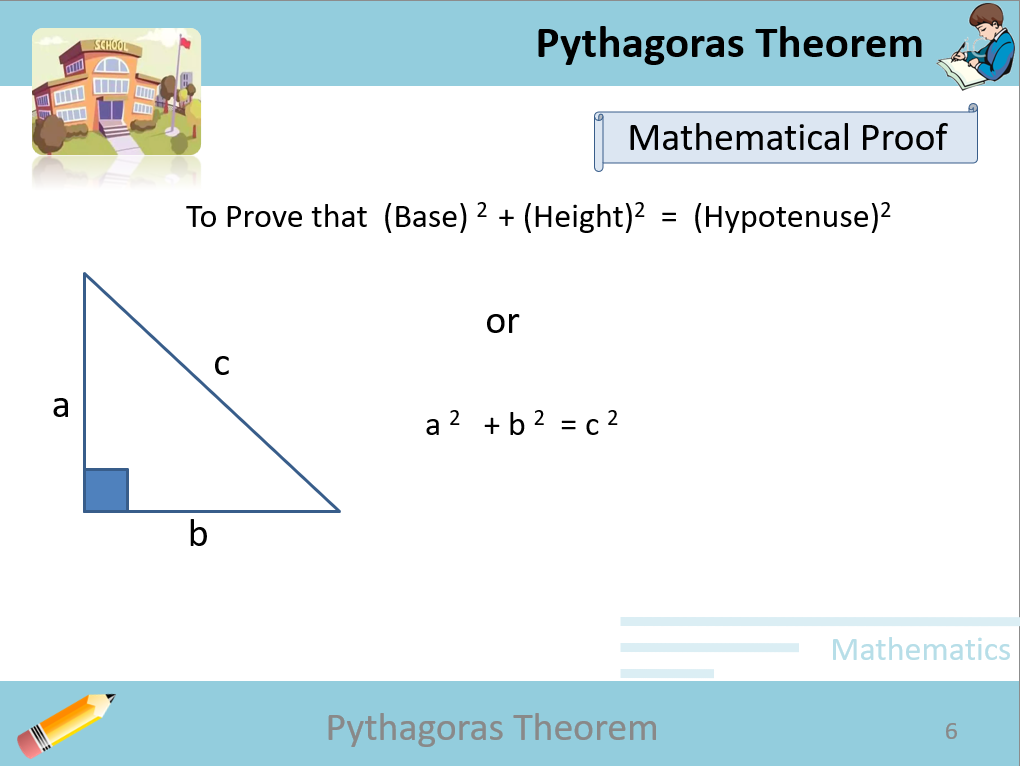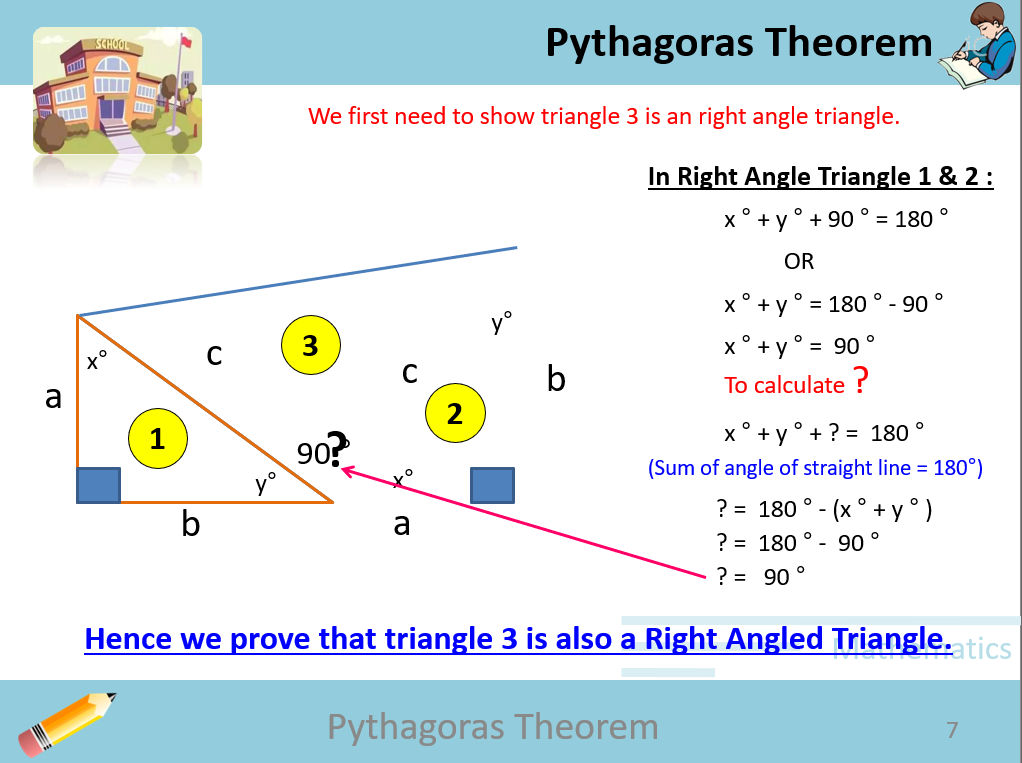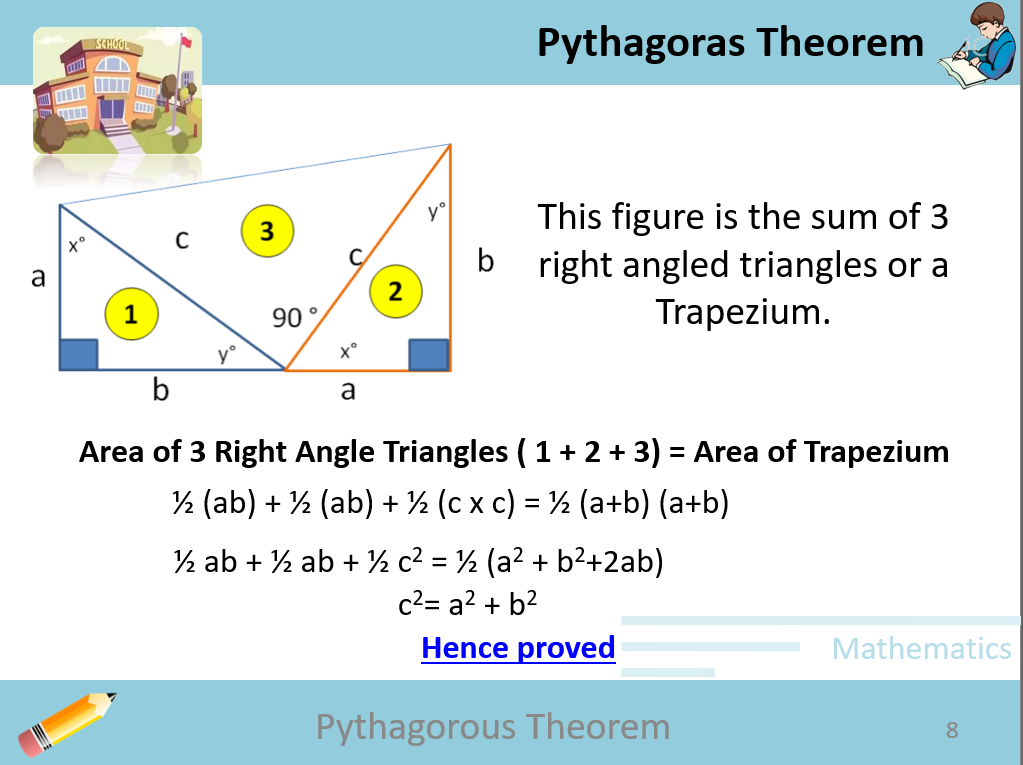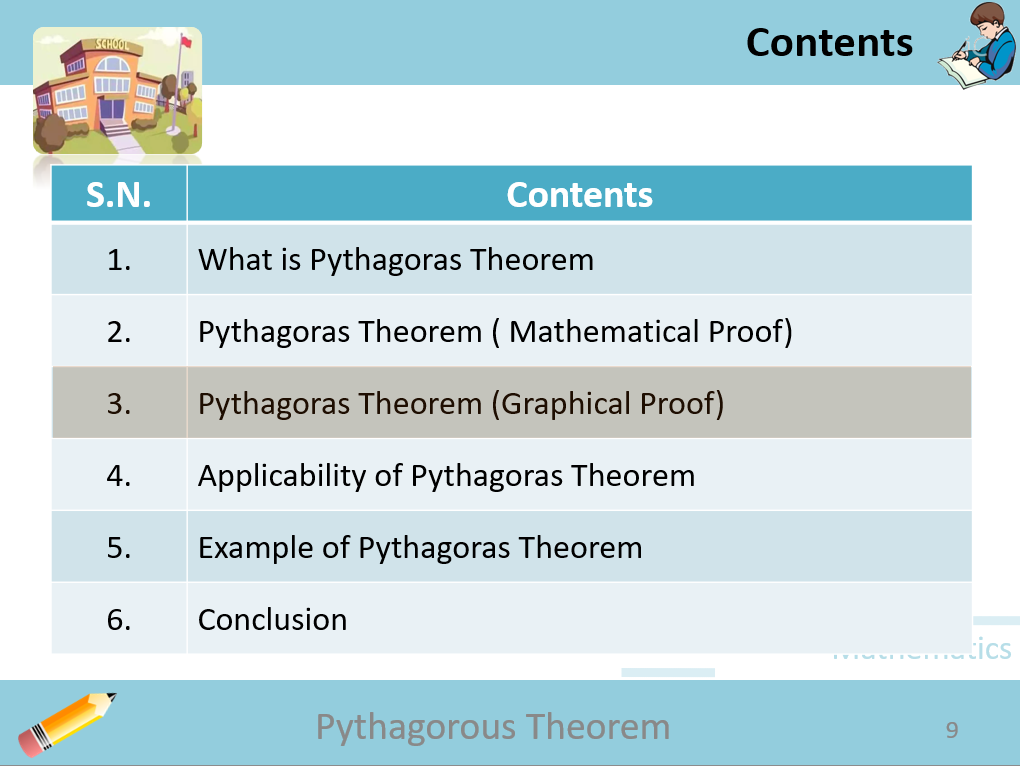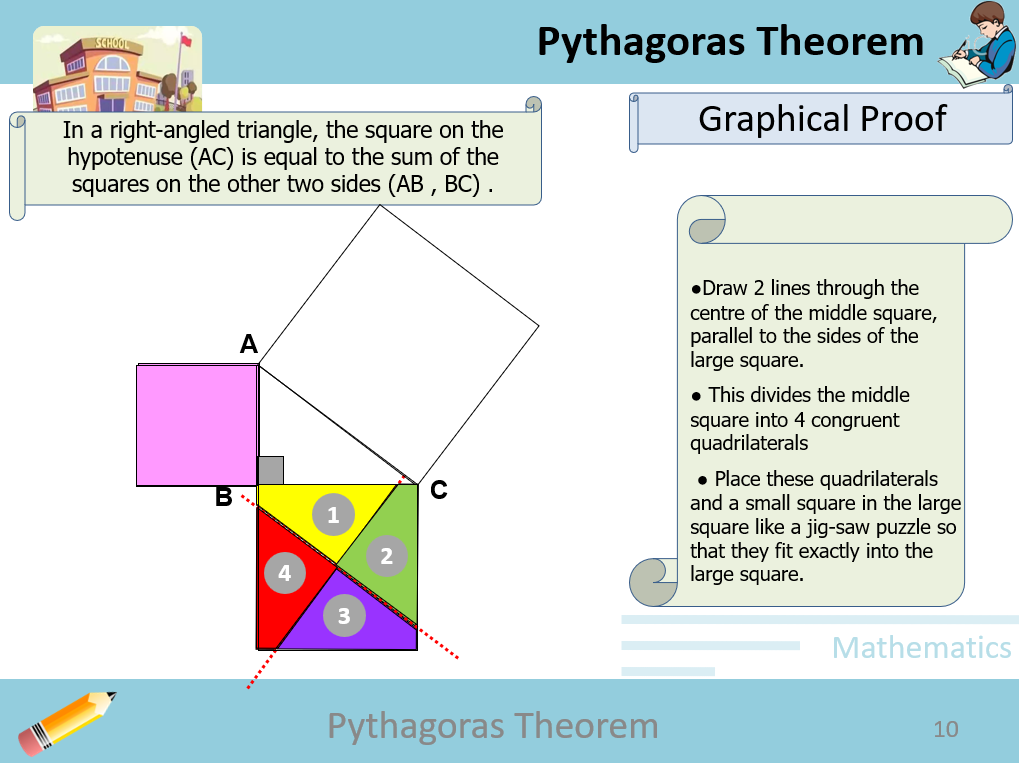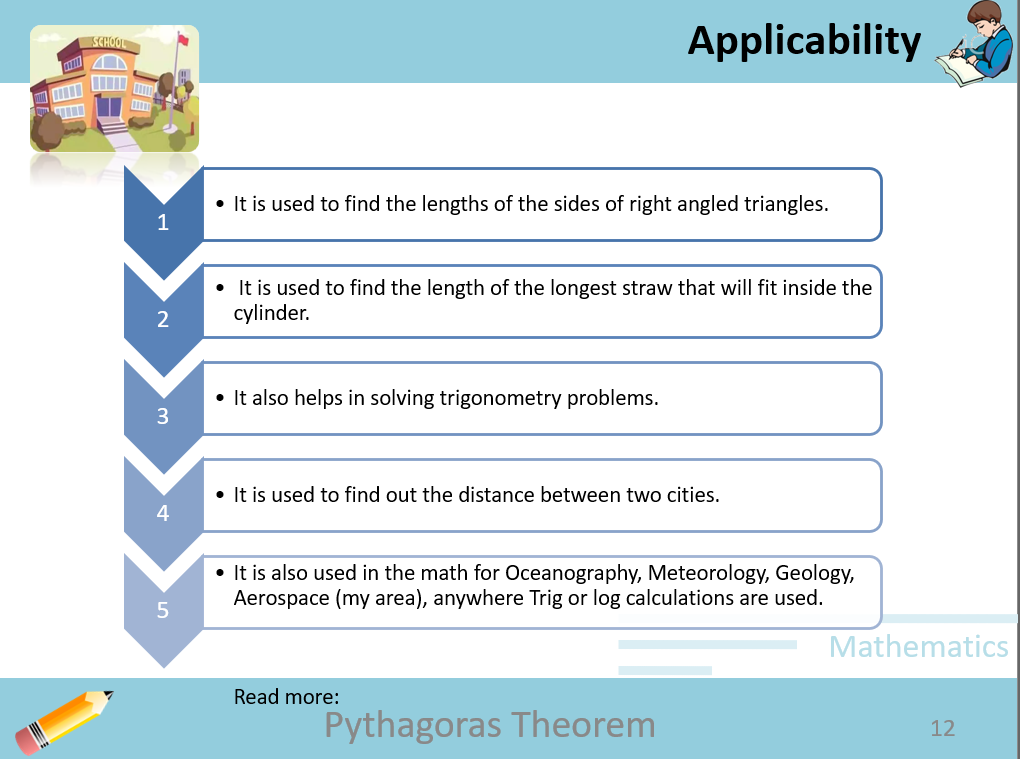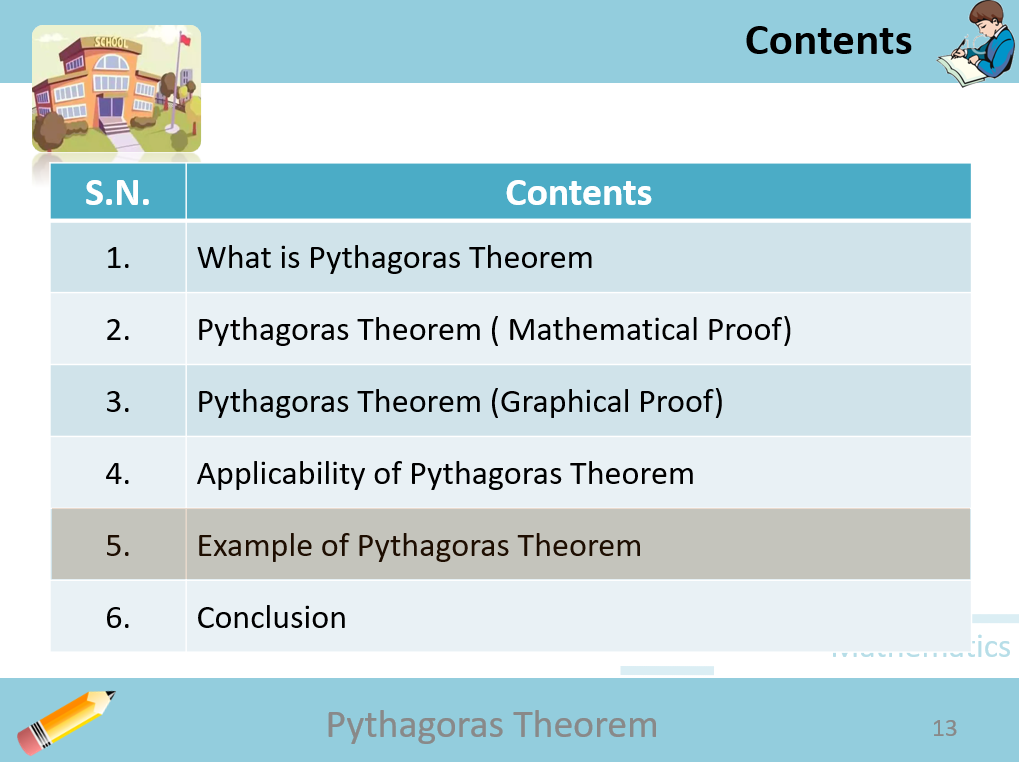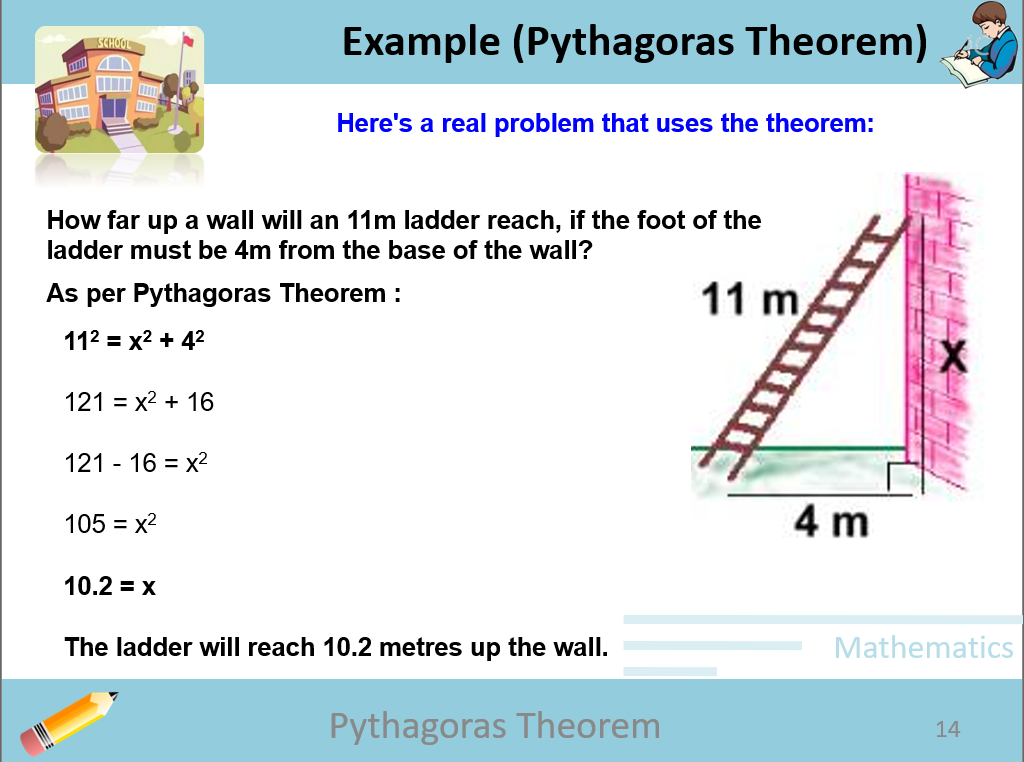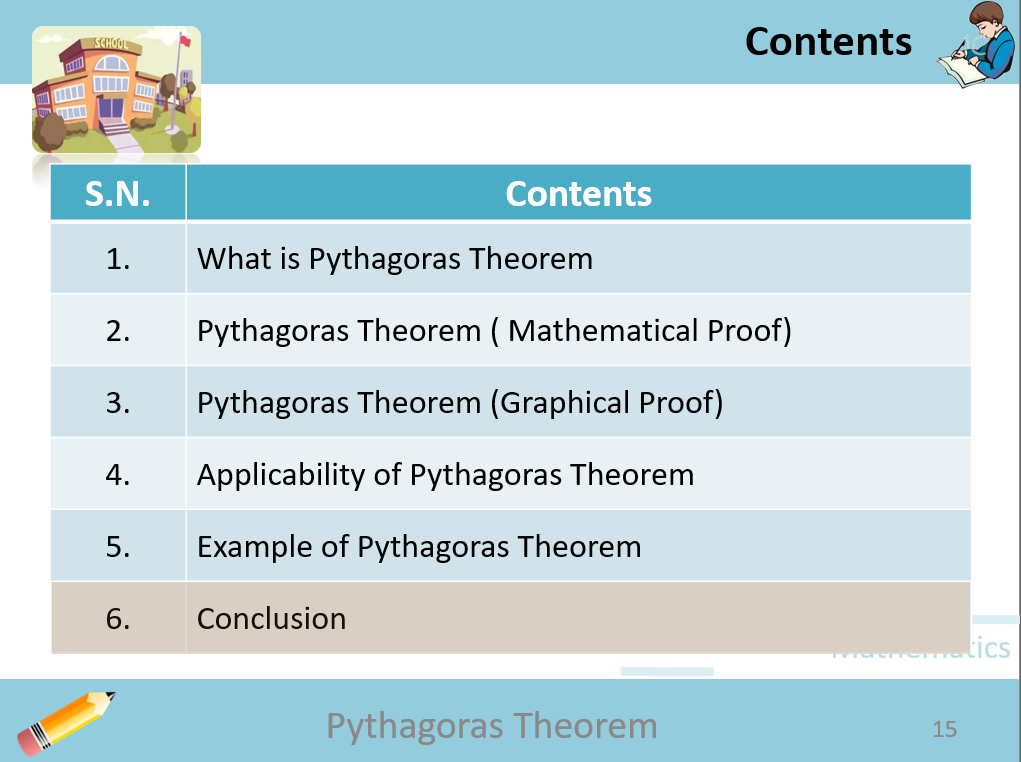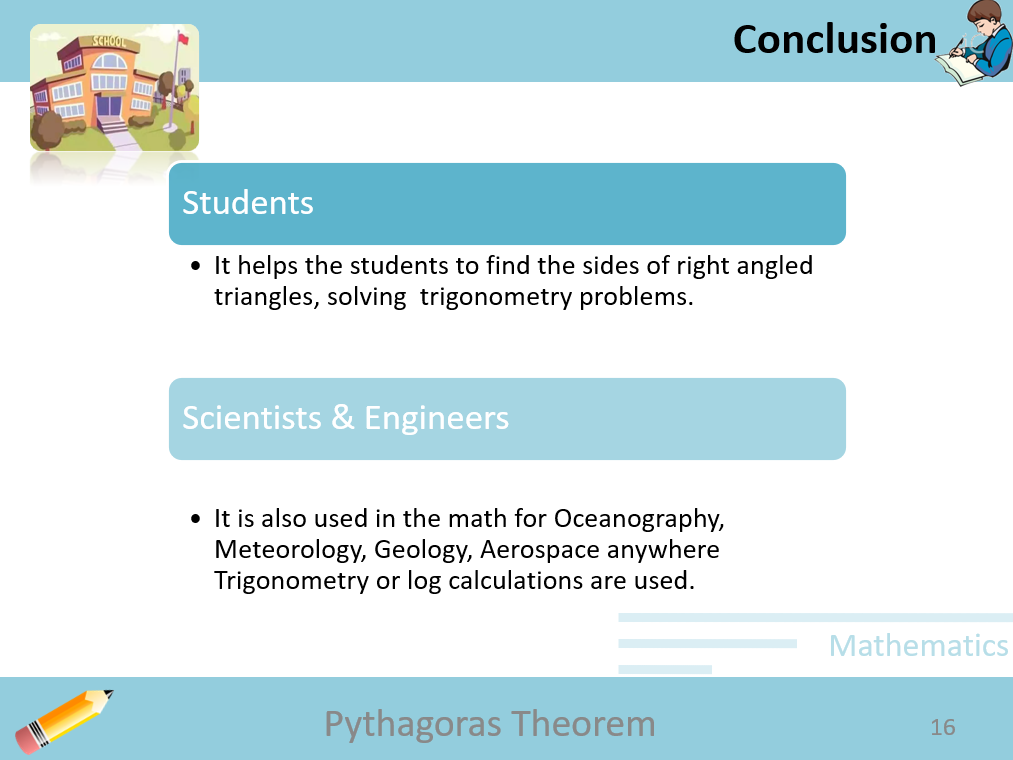Description:

PPT on Pythagoras Theorem

1. Easy to comprehend
2. Integrated animations
3. Learning with Fun

The PPT contains 17 slides (including opening and closing slide)

Topics covered:

• What is Pythagoras Theorem
• Pythagoras Theorem (Mathematical Proof)
• Pythagoras Theorem (Graphical Proof)
• Applicability of Pythagoras Theorem
• Example of Pythagoras Theorem
• Conclusion

Topic: Education Templates, Education & Books,

Color: white, cyan,

tags: animation, mathematics, maths, theorem, fun learning, pythagoras theorem, pythagoras,

• #### 1 200 000+Satisfied Customers

\$20

OR
Topic: Education Templates, Education & Books,

Color: white, cyan,

tags: animation, mathematics, maths, theorem, fun learning, pythagoras theorem, pythagoras,

•••••••••